# Sum If Horizontal – Excel & Google Sheets

This tutorial will demonstrate how to use the SUMIFS Function to sum horizontal data ranges where column headings match specific conditions in Excel and Google Sheets.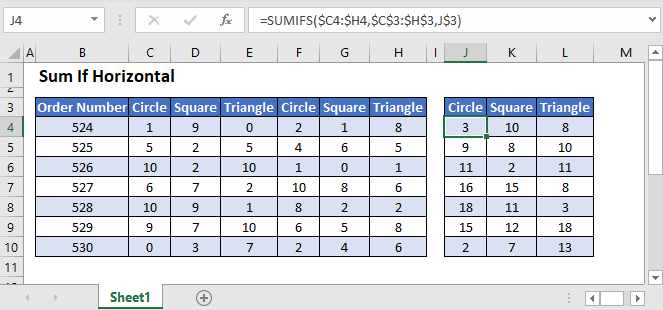## Sum If Using Horizontal Ranges

The SUMIFS Function sums data that meets certain criteria. Its syntax is: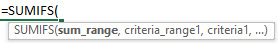This example will sum the number of Products labeled “Circle” for each Order Number by using the SUMIFS Function:

``=SUMIFS(C3:H3,C2:H2,"Circle")``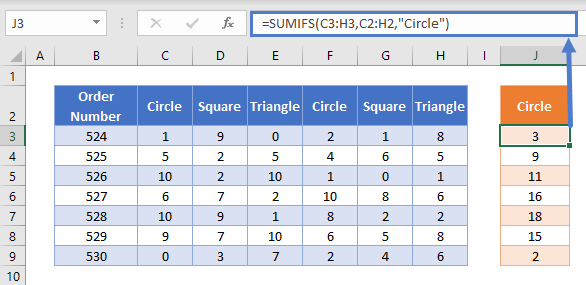The data ranges used in a SUMIFS Function can be any shape or orientation, but they must all be of the exact same dimensions. In the example above, the sum range and the criteria range are each 6 columns wide and 1 row high.

Usually, SUMIFS is used to perform analysis on columns of data, but in this example, we are using horizontal ranges. In the cell J3, we are summing all Products in Order Number 524 in which the column header matches the name “Circle”.

## Sum If Using Horizontal Ranges – Cell References

Usually, it is bad practice to hard-code values into formulas. Instead, it is more flexible to use a separate cell to define the criteria’s value.

We can reference the product shape in the SUMIFS Function to produce a more detailed summary table:

``=SUMIFS(C3:H3,C2:H2,J2)``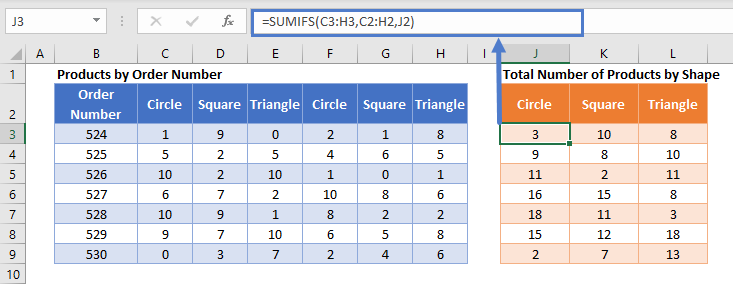If required, we can add summary cells to total all Products by shape using the SUM Function:

``=SUM(J3:J9)``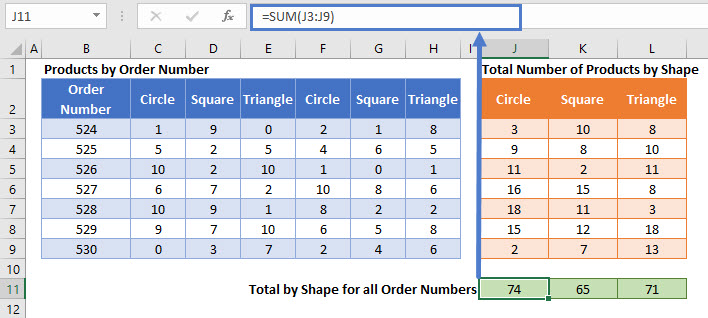## Locking Cell References

To make our formulas easier to read, we’ve shown the formulas without locked cell references:

``=SUMIFS(C3:H3,C2:H2,J2)``

But these formulas will not work properly when copy and pasted elsewhere in your file. Instead, you should use locked cell references like this:

``=SUMIFS(\$C3:\$H3,\$C\$2:\$H\$2,J\$2)``

## Sum If Using 2-Dimensional Data Ranges

We can apply the SUMIFS Function to 2-dimensional data ranges, as long as all input data ranges are the same size.

This example will sum all Scores by Team Name when the input data is shown in 2×5 dimension ranges

``=SUMIFS(E3:F7,B3:C7,H3)``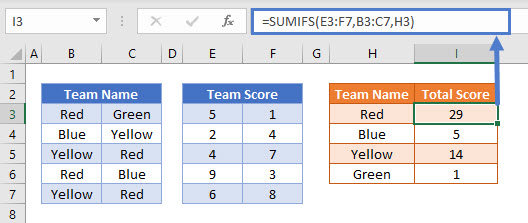This style of using the SUMIFS Function can be hard to follow, so the more standard approach of using single-dimension columns or rows is often preferred.

## Sum If Using Horizontal Ranges in Google Sheets

These formulas work exactly the same in Google Sheets as in Excel.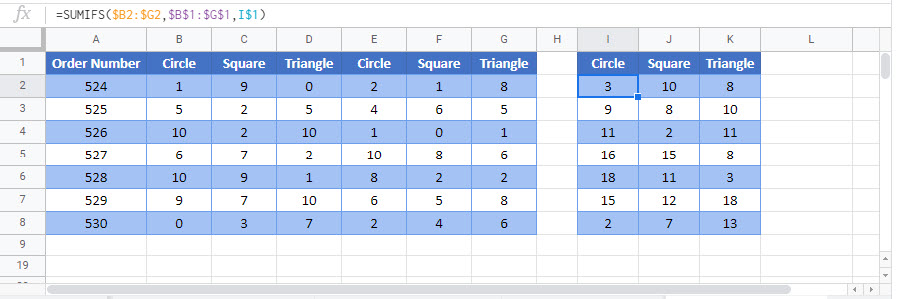### Excel Practice Worksheet

Practice Excel functions and formulas with our 100% free practice worksheets!